## Example Questions

### Example Question #11 : Percentage

What is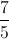as a percentage?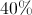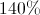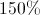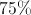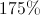Explanation:

Convert the improper fractionto the mixed numeral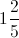or as the decimal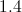.as a percentage is### Example Question #261 : New Sat

A family with 6 children, aged 4, 4, 5, 7, 12, and 13 are moving to a new home. They all want the same bedroom, so the parents have a lottery. Each child places their name in once for every year of age (the four year olds each put their name in 4 times, the seven year old puts his name in 7 times, etc.) What is the probability of the chosen child being 4 years old?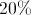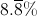It is most likely that the chosen child will be the oldest child.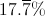Explanation:

First, we will determine the total number of ballots: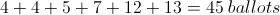Since there are two four year olds, and this question is asking the probability of the chosen child being four, the probability is: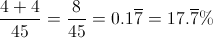### Example Question #11 : Percentage

Three hens each lay 20 eggs for a farmer. Two-fifths of the first hen's eggs are brown. One-fourth of the second hen's eggs are brown. Seven-tenths of the third hen's eggs are white. What percentage of all the eggs are brown?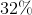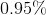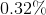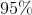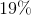Explanation:

The first hen lays 8 brown eggs: 2/5 * 20 = 8

The second lays 5 brown eggs: 1/4 * 20 = 5

The third lays 6 brown eggs: 3/10 * 20 = 6

Add up the brown eggs and divide by 60, the total number of eggs:

19/60 = 0.31666667 = 31.67%, or 32%.

### Example Question #21 : Percentage

What is the closest percent equal to the fraction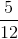?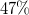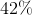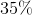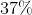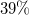Explanation:

The percent is a number over 100.  Set up the proportion to find the unknown value.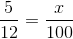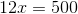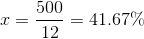The closest answer is.

### Example Question #22 : Percentage

Convert the following fraction to a percent, rounding to the nearest tenth: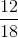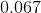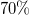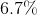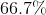Explanation:

Reduce the fraction.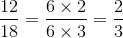Set up the proportion such that some number is over 100.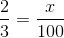Solve for, which is the percent.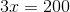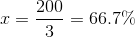### Example Question #23 : Percentage

Express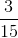as a percent.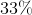Explanation:

To turn this fraction into a percent, let's write it as a decimal first.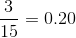Now, let's set up a proportion to make this a percent.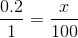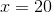Therefore, our answer is